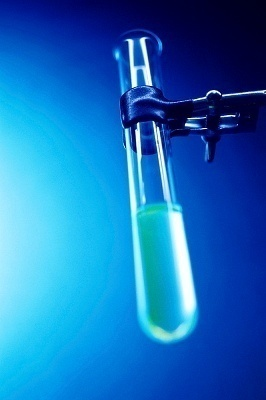•• # How Does the Biuret Test Work?

The biuret test is one in which a researcher can determine whether a mixture has peptide bonds in it or not. By using the biuret test, in seconds an individual knows if they are there. Once they know that the peptide bonds are, in fact, there, they can then attempt to measure the amount of peptide bonds in it by using the Beer-Lambert Law.

## How Does it Work?

A small amount of the mixture that is believed to have peptide bonds in it is added to a test tube and set aside. If five mL of the mixture is used, five mL of a strong base–such as sodium hydroxide or potassium hydroxide–is added to the mixture. Once this has been mixed together, take a few drops of copper(II) sulfate in its aqueous stage and drop them into the test tube. Give the test tube a quick swirl and if the mixture turns purple, that means that there are peptide bonds in the mixture.To determine exactly how much, though, requires use of the Beer-Lambert Law. The Beer-Lambert Law–commonly called Beer's Law–is a law that compares the concentration of the mixture in relation to the absorptivity of the mixture. The equation looks as follows:

### A = a x b x c

In other words, measured absorbance (A) is equal to the wavelength-dependent absorptivity coefficient (a) times the path length (b) times the analyte concentration (c). What is being solved for is c because you were able to measure A. The way to measure A is to take a small amount of the mixture with the base and copper (II) sulfate and put it into a small tube. Then place this into a spectrometer set at 540 nanometers. Take the reading and plug that in for A. The a is constant and can be found in a resource book. Finally, depending on the cell (tube) used determines the size of b. Do the math to find c.

171 queries in 0.484 seconds.